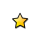On-line GuidesAll GuideseBook StoreiOS / AndroidLinux for BeginnersOffice ProductivityLinux InstallationLinux SecurityLinux UtilitiesLinux VirtualizationLinux KernelSystem/Network AdminProgrammingScripting LanguagesDevelopment ToolsWeb DevelopmentGUI Toolkits/DesktopDatabasesMail SystemsopenSolarisEclipse DocumentationTechotopia.comVirtuatopia.comAnswertopia.comHow To GuidesVirtualizationGeneral System AdminLinux SecurityLinux FilesystemsWeb ServersGraphics & DesktopPC HardwareWindowsProblem SolutionsPrivacy PolicyOpenOffice Calc 3.x Guide
Previous Page Home Next Page

To illustrate a function that accepts arguments, we will write a macro that calculates the sum of its arguments that are positive—it will ignore arguments that are less than zero (see Listing 5).

Listing 5. PositiveSum calculates the sum of the positive arguments.

``` ```

``` Function PositiveSum(Optional x) Dim TheSum As Double Dim iRow As Integer Dim iCol As Integer TheSum = 0.0 If NOT IsMissing(x) Then If NOT IsArray(x) Then If x > 0 Then TheSum = x Else For iRow = LBound(x, 1) To UBound(x, 1) For iCol = LBound(x, 2) To UBound(x, 2) If x(iRow, iCol) > 0 Then TheSum = TheSum + x(iRow, iCol) Next Next End If End If PositiveSum = TheSum End Function ```

The macro in Listing 5 demonstrates a couple of important techniques.

1. The argument x is optional. If the argument is not optional and it is called without an argument, OOo prints a warning message every time the macro is called. If Calc calls the function many times, then the error is displayed many times.
2. IsMissing checks that an argument was passed before the argument is used.
3. IsArray checks to see if the argument is a single value, or an array. For example, =PositiveSum(7) or =PositiveSum(A4). In the first case, the number 7 is passed as an argument, and in the second case, the value of cell A4 is passed to the function.
4. If a range is passed to the function, it is passed as a two-dimensional array of values; for example, =PositiveSum(A2:B5). LBound and UBound are used to determine the array bounds that are used. Although the lower bound is one, it is considered safer to use LBound in case it changes in the future.The macro in Listing 5 is careful and checks to see if the argument is an array or a single argument. The macro does not verify that each value is numeric. You may be as careful as you desire. The more things you check, the more robust the macro is, and the slower it runs.

Passing one argument is as easy as passing two: add another argument to the function definition (see Listing 6). When calling a function with two arguments, separate the arguments with a semicolon; for example, =TestMax(3; -4).

Listing 6. TestMax accepts two arguments and returns the larger of the two.

``` ```

``` Function TestMax(x, y) If x >= y Then TestMax = x Else TestMax = y End If End Function ```

### Arguments are passed as values

Arguments passed to a macro from Calc are always values. It is not possible to know what cells, if any, are used. For example, =PositiveSum(A3) passes the value of cell A3, and PositiveSum has no way of knowing that cell A3 was used. If you must know which cells are referenced rather than the values in the cells, pass the range as a string, parse the string, and obtain the values in the referenced cells.

OpenOffice Calc 3.x Guide
Previous Page Home Next PagePublished under the terms of the Creative Commons License Design by Interspire